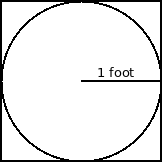## Objective

To get experience writing, compiling and executing simple pthreads programs.

Suppose we toss darts randomly at a square dartboard whose sides are 2 feet in length. Suppose there is also a circle inscribed in the center of the dartboard with a radius of 1 foot, and an area of $\pi$ square feet.

If the darts are uniformly distributed on the dartboard, and we always hit the dartboard, then the number of darts that land inside the circle should satisfy the equation:$\frac{\text{number in circle}}{\text{total number of tosses}} = \frac{\pi}{4}$

since the ratio of the area of the circle to the area of the square is $\frac{\pi}{4}$.

We can use this to estimate the value of $\pi$ with the following algorithm:

1. number in circle = 0
2. do $N$ times:
1. set $x$ to be random number between -1 and 1.
2. set $y$ to be random number between -1 and 1.
3. set distance to be $x \times x + y \times y$.
4. if the distance is less than or equal to 1, increment number in circle.
3. estimate $\pi$ as $4 \times \frac{\text{number in circle}}{N}$

This is called a Monte Carlo method because it uses random sampling.

The larger $N$ is, the more accurate our estimation.

You should write a Pthreads program which uses this method to estimate $\pi$. There are two major ways you could do this:

1. Have each thread do many samples, and send its "number in circle" count to the main thread, which will then add them all together for the final estimate.
2. Have each thread do many samples, and estimate $\pi$, sending the estimate to the main thread which will average them all together.

You can do this either way.

## Details

1. Write a Pthreads program which spawns a constant number of threads (this should be defined at the top of your program).
2. Each thread should do a constant number of tosses (this should be a constant as well).
3. Rather than calling the regular "rand" function, you should call the thread safe version rand_r. This function takes a pointer to the "seed" value. Create a local unsigned int variable for the seed in each thread, and pass its address to rand_r. These also should be given different values for each thread.

4. You can find a random number between -1 and 1 with the expression:

((double) rand_r(&seed) / (double) RAND_MAX) * 2.0 - 1.0
5. The main thread should wait for the child threads to finish, then combine the results.
6. The main thread should then print the final estimate.

When your program works, it should print out a value approximately equal to $\pi$ (e.g. 3.1 - 3.2) when run with suitably large sample size (more than a few thousand total samples).

## Analysis

Test your program with the same number of total tosses, but split into more threads as shown in the following table:
 Threads Tosses Per Thread Estimate Time 1 80,000,000 2 40,000,000 4 20,000,000 8 10,000,000

What is the speedup and efficiency of moving to 8 threads?

Use the "time" command to time your runs:

\$ time ./a.out
Pi is roughly 3.141625

real   0m0.416s
user   0m2.880s
system 0m0.000s


The "real time" is the one we are interested in.

## Submitting

When your program works, email the source code and the analysis to ifinlay@umw.edu.